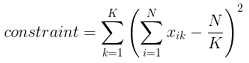# Graph Partitioning with Discrete Quadratic Model

Hi,

I have been working in the past on the graph partitioning problem using a BQM (see e.g. arXiv:1705.03082). For my own education, I was wondering if there is any public example showing how to implement graph partitioning with N > 2 classes using a Discrete Quadratic Model, similarly to what is presented for the graph colouring in the documentation.

Riccardo

• Riccardo,

Just in case you did not find this over the weekend:

https://github.com/dwave-examples/graph-partitioning-dqm

Ed

• Hi Ed,

thanks, indeed I've found it already after some more digging. Perhaps someone can further explain what happens behind the scenes. I understand that in DQM, each n-dim variable is actually an array of n qubits (q0 q1 ... qn), but I'm not sure how the overall assignment to one class is done. How does the system enforces that e.g. only the 2nd qubit is 1 and all the others are 0? Or is it repeated multiple times, and the class is obtained by a majority vote? If you implement something similar with a BQM, sometimes you have "invalid" solutions such as (1, 0, ...,1). I also discussed this point with the authors of the paper above and they confirmed there's no obvious way to avoid this situation with a BQM, so I hope the DQM helps here in some non-trivial way.

Cheers,
Riccardo

• Hi Riccardo,

Apologies for the delayed response!

DQM solver has one-hot implementation which means for each variable it enforces that only one case will be 1 and rest will be 0.

For your other point, DQM does not handle the valid/invalid solution implicitly. Similar to the BQM implementation, the constraint defined in DQM example(mentioned by Ed above) tries to enforce that all the partitions have equal size.There is still a possibility that returned solution is invalid(unequal partitions).

Both the implementations return output in different formats. Graph partitioning using BQM returns only valid/invalid status and number of edges between valid partitions, where as DQM implementation also returns the partitions and number of nodes in each partition giving the user more information. If you want to include an output for valid/invalid solutions to your DQM example, you might need to add a similar check as used in BQM implementation.

Also, if you are interested in learning more about DQM, you might find our latest webinar Moving Beyond Binary: Exploring the Discrete Quadratic Model helpful. This goes over the problem formulation using DQM in detail.